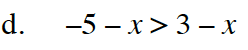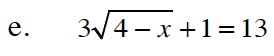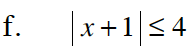### Home > CC4 > Chapter 11 > Lesson 11.2.1 > Problem11-53

11-53.
1. Solve the inequalities and equations below. Represent your solutions for each inequality on a number line. Homework Help ✎

2.  a.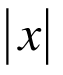+ 3 < 5 b. 5(2x + 1) ≥ 30 c.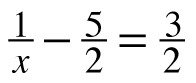d. −5 − x > 3 − x e. 3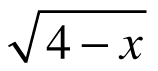+ 1 = 13 f.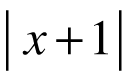≤ 4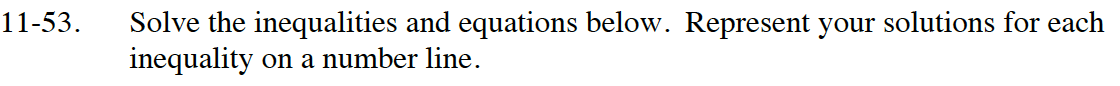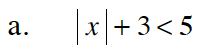Isolate the absolute value by subtracting 3 from both sides.
|x| + 3 < 5
|x| + 3 − 3 < 5 − 3
|x| < 2

Change the inequality into an equation and solve for x.
|x| < 2
|x| = 2
x = {2, −2}

Graph both values of x on the number line, which divides the line into three regions.
Substitute a value from each region into the original inequality.
Whichever regions make the inequality true are the solutions.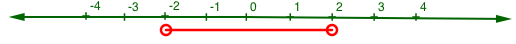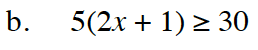Change to an equation and solve for x.
5(2x + 1) ≥ 30
5(2x + 1) = 30
x = 2.5

Graph x on a number line.
Then check values from both regions to find the solution.

x ≥ 2.5
Don't forget to represent your solution with a number line!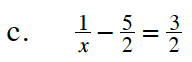Multiply both sides by 2x as Fraction Buster to eliminate the fractions.

$\left(\frac{1}{x}\right)2x-\left(\frac{5}{2}\right)2x=\left(\frac{3}{2}\right)2x$

2 − 5x = 3x

$x=\frac{1}{4}$